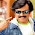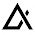### E.M.F Equation of aTransformer

When the primary winding is excited by an alternating voltage V1, it circulates alternating current, producing an alternating flux Φ. The primary winding has N1 number of turns. The alternating flux Φ linking with the primary winding itself induces an e.m.f in it denoted as E1. The flux links with secondary winding through the common magnetic core. It produces induced e.m.f. E in the secondary winding. This is mutually induced e.m.f. Let us derive the equations for E1 and E2.
The primary winding is excited by purely sinusoidal alternating voltage. Hence the flux produced is also sinusoidal in nature having maximum value of Φm as show in the Fig. 1.Fig.  1  Sinusoidal flux
The various quantities which affect the magnitude of the induced e.m.f. are :
Φ = Flux
Φm = Maximum value of flux
N1 = Number of primary winding turns
N2 = Number of secondary winding turns
f = Frequency of the supply voltage
E1 = R.M.S. value of the primary induced e.m.f.
E2 = R.M.S. value of the secondary induced e.m.f.
From Faraday's law of electromagnetic induction the voltage e.m.f. induced in each turn is proportional to the average rate of change of flux.
...      average e.m.f. per turn = average rate of change of flux
...      average e.m.f. per turn = dΦ/dt
Now                 dΦ/dt = Change in flux/Time required for change in flux
Consider the 1/4 th cycle of the flux as shown in the Fig.1. Complete cycle gets completed in 1/f seconds. In 1/4 th time period, the change in flux is from 0 to Φm.
...                  dΦ/dt = (Φm - 0)/(1/4f)           as dt for 1/4 th time period is 1/4f seconds
= 4 f  Φm       Wb/sec
...    Average e.m.f. per turn =  4 f Φm   volts
As is sinusoidal, the induced e.m.f. in each turn of both the windings is also sinusoidal in nature. For sinusoidal quantity,
From factor = R.M.S. value/Average value = 1.11
...    R.M.S. value of induced e.m.f. per turn
= 1.11 x 4 f Φm = 4.44 f Φm
There are number of primary turns hence the R.M.S value of induced e.m.f. of primary denoted as is E1,
E1 = N1 x 4.44 f  Φm      volts
While as there are number of secondary turns the R.M.S values of induced e.m.f. of secondary denoted is E2 is,
E2 = N2 x 4.44 f Φm         volts
The expression of E1 and E2 are called e.m.f. equation of  a transformer.
Thus e.m.f. equations are,
E1 = 4.44 f ΦN1              volts             ............(1)
E2 = 4.44 f  Φ N2             volts             .............(2)

1.2.3.4.